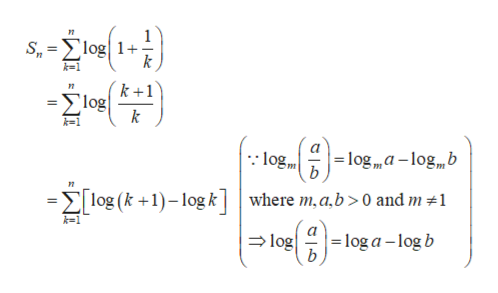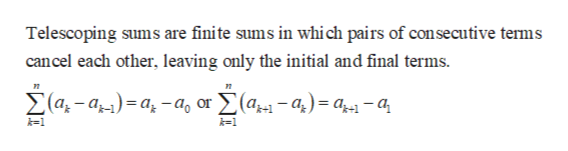# Real AnalysisI posted this question earlier and got a response . I have further questions on the response.  I am including the URL of the previous response:https://www.bartleby.com/questions-and-answers/real-analysis-does-the-series-log11n-from-n1-to-infinity-converge-or-diverge-prove-the-convergence-o/97a36976-4bf8-4a81-bf97-1b9c2d442972My original question was:Prove that the series Sum of log(1+1/n) from n=1 to infinity converges or diverges.The questions I have on the response are as follows:1.  Why can I just change from log to ln? I seem to recall something about ln and log being interchangeable despite that log is log base 10 and ln is log base e.2.  Why does the sequence of partial sums become (ln(2)-ln(1))+(ln(3)-ln(2))+(ln(n)-ln(n-1))+(ln(n+1)-ln(n))=ln(n+1).I need a lot more detail.  I seem to be incredibly dense with Real Analysis, despite earning top grades in my previous classes.  There are always parts of the proofs that I do not understand which prevents me from understanding the material.  Thank you.

Question
3 views

Real Analysis

I posted this question earlier and got a response . I have further questions on the response.  I am including the URL of the previous response:

My original question was:

Prove that the series Sum of log(1+1/n) from n=1 to infinity converges or diverges.

The questions I have on the response are as follows:

1.  Why can I just change from log to ln? I seem to recall something about ln and log being interchangeable despite that log is log base 10 and ln is log base e.

2.  Why does the sequence of partial sums become (ln(2)-ln(1))+(ln(3)-ln(2))+(ln(n)-ln(n-1))+(ln(n+1)-ln(n))=ln(n+1).

I need a lot more detail.  I seem to be incredibly dense with Real Analysis, despite earning top grades in my previous classes.  There are always parts of the proofs that I do not understand which prevents me from understanding the material.  Thank you.

check_circle

Step 1

1.

The general logarithm form is, loga x.

Suppose the base a = e , the logarithm form is, loge x which is called as natural logarithm.

Usually, the natural logarithm can be written as ‘ln x’.

Suppose the base a = 10 , the logarithm form is, log10 x which is called as common logarithm.

Usually, the common logarithm can be written as ‘log x’.

Clearly, log x and ln x are not same.

So, keep the sequence as it is.

Step 2

2.

The n-th partial sum of the series is,help_outlineImage TranscriptioncloseS log1+- k n Σlog! k log log,,a-log,b Elog (k1)log kwhere m,a,b > 0 and m 1 k-1 loga-logb log b fullscreen
Step 3

Known resu...help_outlineImage TranscriptioncloseTelescoping sums are finite sums in whi ch pairs of consecutive terms can cel each other, leaving only the initial and final terms a-a)=a-a0 or (a*-a,)= a- a k1 k1 fullscreen

### Want to see the full answer?

See Solution

#### Want to see this answer and more?

Solutions are written by subject experts who are available 24/7. Questions are typically answered within 1 hour.*

See Solution
*Response times may vary by subject and question.
Tagged in

### Math